FreeBuf.COM网络安全行业门户，每日发布专业的安全资讯、技术剖析。FreeBuf+小程序

IDAPython：让你的生活更美好（五） 金币
2016-01-19 07:00:02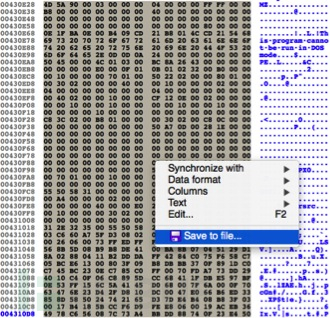```1. 我们必须在IDA环境中用PIP安装第三方python链接库
2. 已经标识出了内嵌代码
3. 需要计算要提取的可执行代码的大小```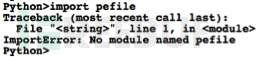```import sys
print sys.path

Result:
['/System/Library/Frameworks/Python.framework/Versions/2.7/lib/python27.zip', '/System/Library/Frameworks/Python.framework/Versions/2.7/lib/python2.7', '/System/Library/Frameworks/Python.framework/Versions/2.7/lib/python2.7/plat-darwin', '/System/Library/Frameworks/Python.framework/Versions/2.7/lib/python2.7/plat-mac', '<Truncated>']```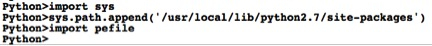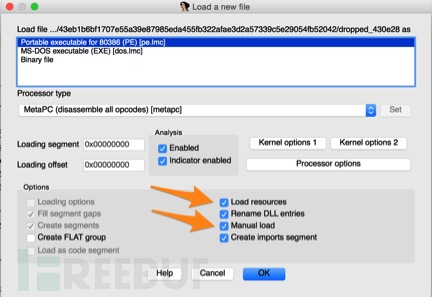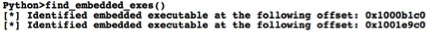```def find_string_occurrences(string):
results = []
base = idaapi.get_imagebase() + 1024
while True:
ea = FindBinary(base, SEARCH_NEXT|SEARCH_DOWN|SEARCH_CASE, '"%s"' % string)
if ea != 0xFFFFFFFF:
base = ea+1
else:
break
results.append(ea)
return results```

```def find_embedded_exes():
results = []
exes = find_string_occurrences("!This program cannot be run in DOS mode.")
if len(exes) > 1:
for exe in exes:
m = Byte(exe-77)
z = Byte(exe-76)
if m == ord("M") and z == ord("Z"):
mz_start = exe-77
print "[*] Identified embedded executable at the following offset: 0x%x" % mz_start
results.append(mz_start)
return results``````def calculate_exe_size(begin):
buff = ""
for c in range(0, 1024):
buff += chr(Byte(begin+c))
pe = pefile.PE(data=buff)
total_size = 0
# Iterate through each section and add section size
for section in pe.sections:
total_size += section.SizeOfRawData
return total_size```

```def extract_exe(name, begin, size):
buff = ""
for c in range(0, size):
buff += chr(Byte(begin+c))
f = open(name, 'wb')
f.write(buff)
f.close()```

https://github.com/pan-unit42/public_tools/blob/master/ida_scripts/idapython_pt5.py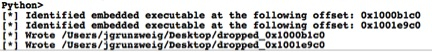*原文地址：Paloaltonetworks，东二门陈冠希/编译，转载请注明来自FreeBuf黑客与极客（FreeBuf.COM）

# python # IDA # 逆向分析

+ 收入我的专辑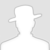• 0 文章数
• 0 评论数
• 0 关注者